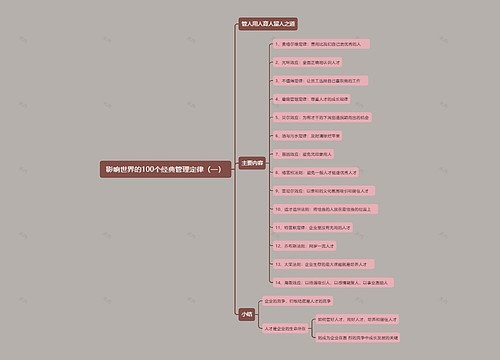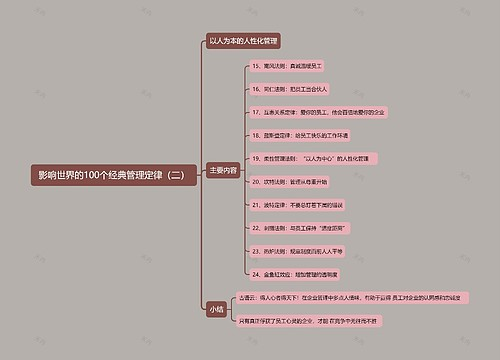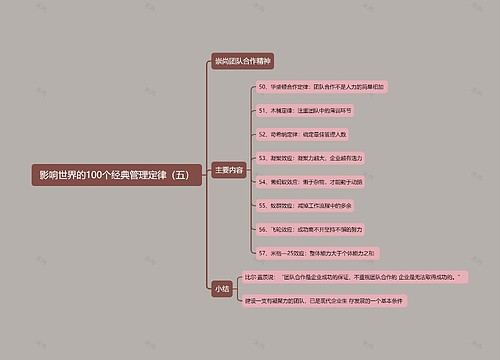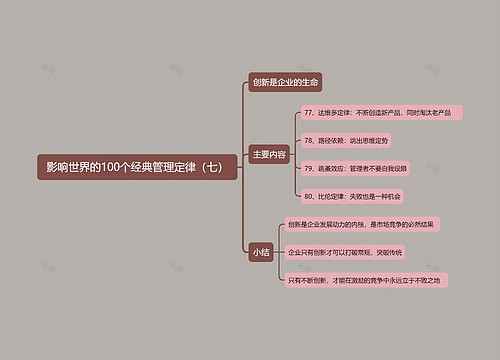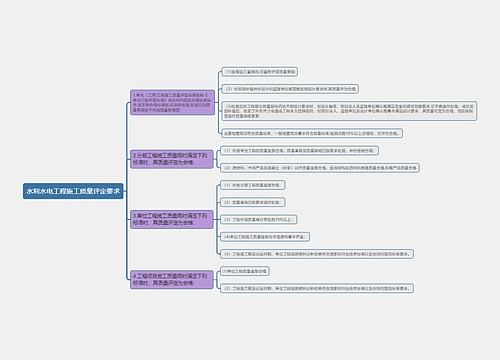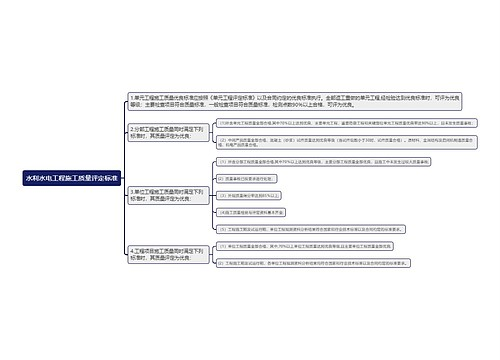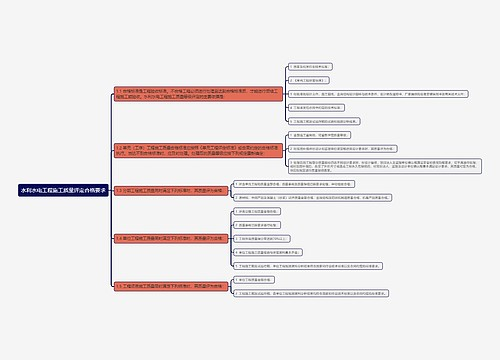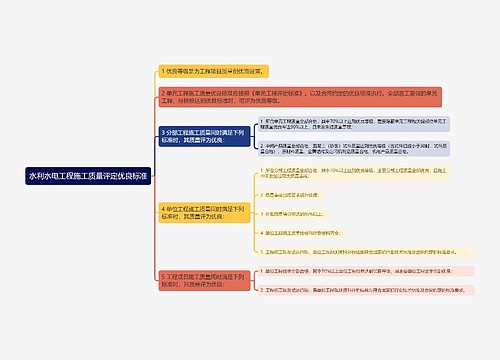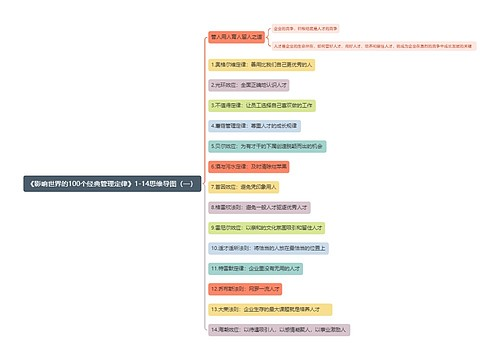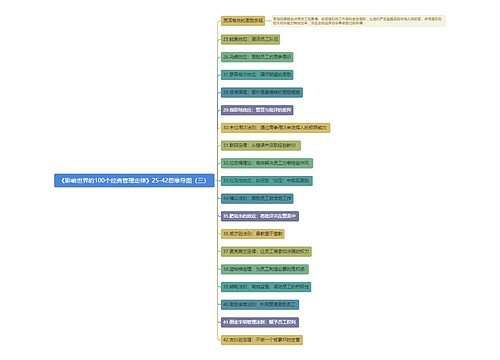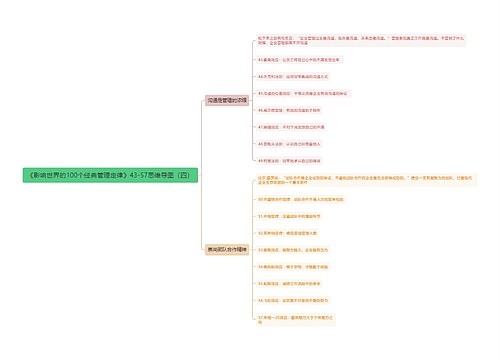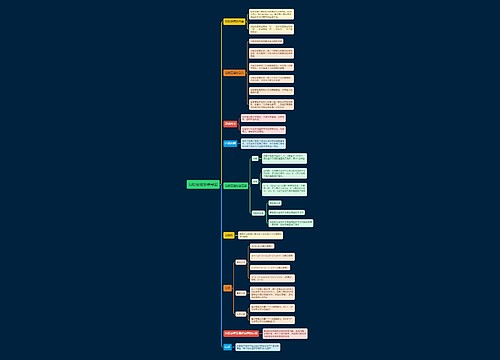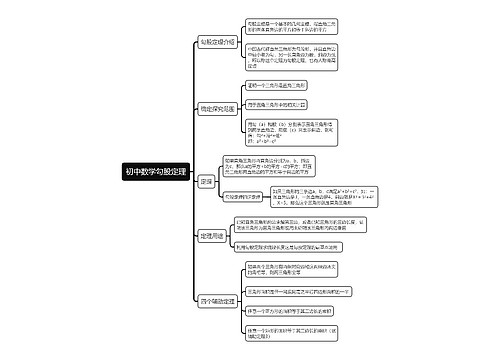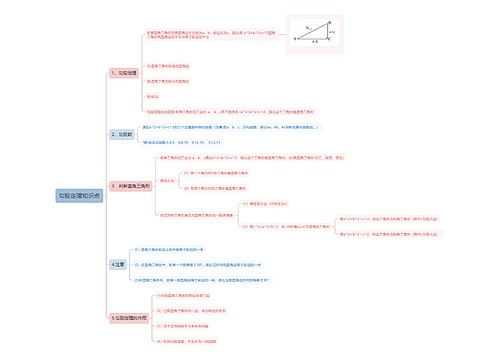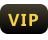成为

# 定积分的性质思维导图

收藏
分享## 思维导图大纲

• 定积分的性质思维导图模板大纲

1. ## “定积分”的简单性质

1. 性质1：设a与b均为常数，则∫(a->b)[a*f(x)+b*g(x)]dx=a*∫(a->b)f(x)dx+b*∫(a->b)g(x)dx。

2. 性质2：设a<c<b,则∫(a->b)f(x)dx=∫(a->c)f(x)dx+f(c->b)f(x)dx。

3. 性质3：如果在区间【a,b】上f(x)恒等于1,那么∫(a->b)1dx=∫(a->b)dx=b-a。

4. 性质4：如果在区间【a,b】上f(X)>=0,那么∫(a->b)f(x)dx>=0(a<b)。

5. 性质5：设M及m分别是函数f(x)在区间【a,b】上的最大值和最小值，则m(b-a)<=∫(a->b)f(x)dx<=M(b-a)(a<b)。

6. 性质6（定积分中值定理）：如果函数f(x)在积分区间【a,b】上连续，那么在【a,b】上至少存在一个点c，使得∫(a->b)f(x)dx=f(c)(b-a)(a<=c<=b)成立。

7. 性质7：若a>b则∫_a^bf(x)=-∫_b^af(x)。

2. ## 定积分

1. 定积分是积分的一种，是函数f(x)在区间[a,b]上积分和的极限。

2. 一个函数，可以存在不定积分，而不存在定积分；也可以存在定积分，而不存在不定积分。一个连续函数，一定存在定积分和不定积分；若只有有限个间断点，则定积分存在；若有跳跃间断点，则原函数一定不存在，即不定积分一定不存在。

## 相关思维导图模板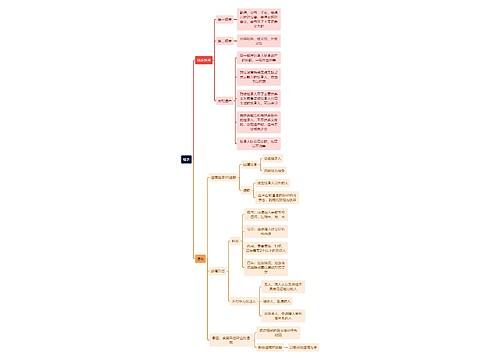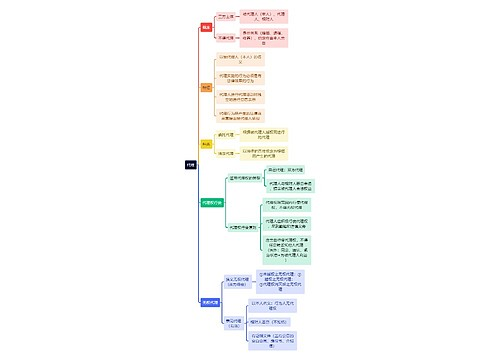### 相关思维导图专辑